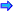# Lecture 2 ExamplesOpen table as spreadsheet

Program

Demonstrates

This program received the student name, the number of grades to average and the value of each grade. It then calculates the average of the grades and displays the student name and average.

functions.h

This program received the student name, the number of grades to average and the value of each grade. It then calculates the average of the grades and displays the student name and average. It works with the file: functions.h.

EXAMPLE2C.CPP

This program uses the concept of namespace to simplify the coding.

fibonacci.cpp

This program demonstrates the use of a for( ) loop to repeat a process.` /*       program_id    grades.cpp       written_by    don voils       date_written  5/3/2006       program_description This program received the student name,                 the number of grades to average and the                 value of each grade. It then calculates                 the average of the grades and displays                 the student name and average. */     #include<iostream>     #include<string>     using namespace std;     double average(short number);     int main()     {       string name;       short number_grades;       double grade;       cout << endl << "What is the student's last name? ";       cin >> name;       cout << endl << "How many grades need to be averaged? ";       cin >> number_grades;       grade = average(number_grades);       cout << "Student " << name << " had an average grade of "          << grade << endl << endl;       char resp;       cout << endl << endl << "Continue? ";       cin >> resp;       return 0;     }     double average(short number)     {      short index;      double sum,score;      sum = 0;      for(index=1;index <= number; ++index)      {        cout << endl << "What was score number "<< index << "? ";        cin >> score;        sum = sum + score;      }      return sum/number;    } // program_id    functions.h // written_by    don voils // date_written 3/2/2006 // description   This header file is called by the program //                grades2.cpp // double average(short number) {   short index;   double sum,score;   sum = 0;   for(index=1;index <= number; ++index)   {     cout << endl << "What was score number "<< index << "? ";     cin >> score;     sum = sum + score;   }   return sum/number; } /*        program_id     grades2.cpp        written_by    don voils        date_written  1/2/2007        program_description This program received the student name,                  the number of grades to average and the                  value of each grade. It then calculates                  the average of the grades and displays                  the student name and average. */ #include<iostream> #include<string> using namespace std; #include "functions.h" int main() {    string name;    short number_grades;    double grade;    cout << endl << "What is the student's last name? ";    cin >> name;    cout << endl << "How many grades need to be averaged? ";    cin >> number_grades;    grade = average(number_grades);    cout << "Student " << name << " had an average grade of "       << grade << endl << endl;    char resp;    cout << endl << endl << "Continue? ";    cin >> resp;    return 0; } //  program_id   exampl2c.cpp //  written_by   don voils //  date_written 2/4/2006 //  program_description  This program uses the concept of //              namespace to simplify the coding. // #include<iostream> #include<iomanip> using namespace std; int main() {     const double factor = 1.5;     double payRate;     double regularHours;     double overtimeHours;     double totalPay;     double regularPay;     double overtimePay;     cout << endl << "What is the employee's regular pay rate? ";     cin >> payRate;     cout << endl << "What is the employee's regular hours? ";     cin >> regularHours;     cout << endl << "What is the employee's overtime hours? ";     cin >> overtimeHours;     regularPay = regularHours * payRate;     overtimePay = overtimeHours * payRate * factor;     totalPay = regularPay + overtimePay;     cout << endl << "The regular hours was " << regularHours;     cout << endl << "The overtime hours was " << overtimeHours;     cout << fixed << setprecision(2);     cout << endl << "The regular pay was \$" << regularPay;     cout << endl << "The overtime pay was \$"<< overtimePay;     cout << endl << "The total pay was \$"<< totalPay << endl << endl;     return 1; } //program_id     fibonacci.cpp //written_by    don voils //date_written  4/2/2006 // //description   This program demonstrates the use of //              a for() loop to repeat a process. // #include<iostream> using namespace std; void main() {    short index;    long first, second, third;    first = 0L;    second = 1L;    for(index = 3;index <= 10; ++index)    {      third = first + second;      first = second;      second = third;    }    cout << "The tenth Fibonacci number is " << third << endl << endl; } `